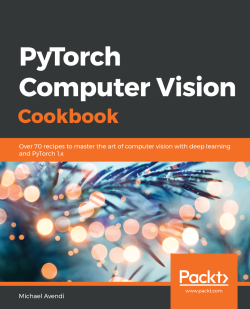### PyTorch Computer Vision Cookbook

By Michael Avendi
• Constantly updated with 100+ new titles each month
• Breadth and depth in over 1,000+ technologies

Computer vision techniques play an integral role in helping developers gain a high-level understanding of digital images and videos. With this book, you’ll learn how to solve the trickiest problems in computer vision (CV) using the power of deep learning algorithms, and leverage the latest features of PyTorch 1.x to perform a variety of CV tasks.

Starting with a quick overview of the PyTorch library and key deep learning concepts, the book then covers common and not-so-common challenges faced while performing image recognition, image segmentation, object detection, image generation, and other tasks. Next, you’ll understand how to implement these tasks using various deep learning architectures such as convolutional neural networks (CNNs), recurrent neural networks (RNNs), long short-term memory (LSTM), and generative adversarial networks (GANs). Using a problem-solution approach, you’ll learn how to solve any issue you might face while fine-tuning the performance of a model or integrating it into your application. Later, you’ll get to grips with scaling your model to handle larger workloads, and implementing best practices for training models efficiently.

By the end of this CV book, you’ll be proficient in confidently solving many CV related problems using deep learning and PyTorch.

Publication date:
March 2020
Publisher
Packt
Pages
364
ISBN
9781838644833

# Getting Started with PyTorch for Deep Learning

There has been significant progress in computer vision because of deep learning in recent years. This helped to improve the performance of various tasks such as image recognition, object detection, image segmentation, and image generation. Deep learning frameworks and libraries have played a major role in this process. PyTorch, as a deep learning library, has emerged since 2016 and gained great attention among deep learning practitioners due to its flexibility and ease of use.

There are several frameworks that practitioners use to build deep learning algorithms. In this book, we will use the latest version of PyTorch 1.0 to develop and train various deep learning models. PyTorch is a deep learning framework developed by Facebook's artificial intelligence research group. It provides flexibility and ease of use at the same. If you are familiar with other deep learning frameworks, you will find PyTorch very enjoyable.

In this chapter, we will provide a review of deep learning concepts and their implementation using PyTorch 1.0. We will cover the following recipes:

• Installing software tools and packages
• Working with PyTorch tensors
• Building models
• Defining the loss function and optimizer
• Training and evaluation

Developing deep learning algorithms is comprised of two steps: training and deployment. In the training step, we use training data to train a model or network. In the deployment step, we deploy the trained model to predict the target values for new inputs.

To train deep learning algorithms, the following ingredients are required:

• Training data (inputs and targets)
• The model (also called the network)
• The loss function (also called the objective function or criterion)
• The optimizer

You can see the interaction between these elements in the following diagram: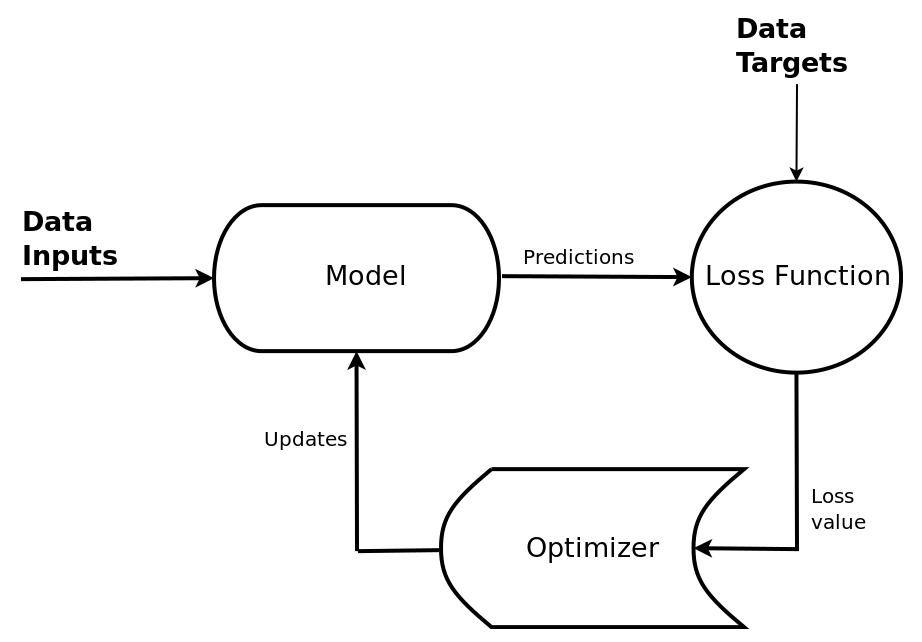The training process for deep learning algorithms is an iterative process. In each iteration, we select a batch of training data. Then, we feed the data to the model to get the model output. After that, we calculate the loss value. Next, we compute the gradients of the loss function with respect to the model parameters (also known as the weights). Finally, the optimizer updates the parameters based on the gradients. This loop continues. We also use a validation dataset to track the model's performance during training. We stop the training process when the performance plateaus.

# Technical requirements

It is assumed that you are familiar with deep learning and computer vision concepts. In addition, you are expected to have medium proficiency in Python programming.

Deep learning algorithms are computationally heavy. You will need a computer with decent GPU hardware to build deep learning algorithms in a reasonable time. The training time depends on the model and data size. We recommend equipping your computer with an NVIDIA GPU or using services such as AWS to rent a computer on the cloud. Also, make sure to install the NVIDIA driver and CUDA. For the rest of this book, whenever referring to GPU/CUDA, we assume that you have installed the required drivers. You can still use your computer with a CPU. However, the training time will be much longer and you'll need to be patient!

# Installing software tools and packages

We will use Python, PyTorch, and other Python packages to develop various deep learning algorithms in this book. Therefore, first, we need to install several software tools, including Anaconda, PyTorch, and Jupyter Notebook, before conducting any deep learning implementation.

# How to do it...

In the following sections, we will provide instructions on how to install the required software tools.

# Installing Anaconda

Let's look at the following steps to install Anaconda:

1. To install Anaconda, visit the following link: https://www.anaconda.com/distribution/.
2. In the link provided, you will find three distributions: Windows, macOS, and Linux. Select the desired distribution. You can download either Python 2.x or Python 3.x. We recommend using a Linux system and downloading and installing Python 3.x version.
3. After installing Anaconda, create a conda environment for PyTorch experiments. For instance, in the following code block, we create conda-pytorch as follows:
`# choose your desired python version\$ conda create env conda-pytorch python=3.6`
1. Activate the environment on Linux/Mac using the following command:
`# activate conda environment on Linux/mac\$ source activate conda-pytorch# After activation, (conda-pytorch) will be added to the prompt.`
1. Activate the environment on Windows using the following command:
`# activate conda environment on Windows\$ activate conda-pytorch# After activation, (conda-pytorch) will be added to the prompt.`

In the next section, we will show you how to install PyTorch.

# Installing PyTorch

Now, let's look at the installation of PyTorch:

1. To install PyTorch, click on the following link: https://pytorch.org/.
2. Scroll down to the Quick Start Locally section. From the interactive table, select the options that are appropriate for your computer system.
3. For instance, if we would like to install PyTorch 1.0 on a Linux OS using the Conda package, along with Python 3.7 and CUDA 9.0, we can make the selections shown in the following screenshot: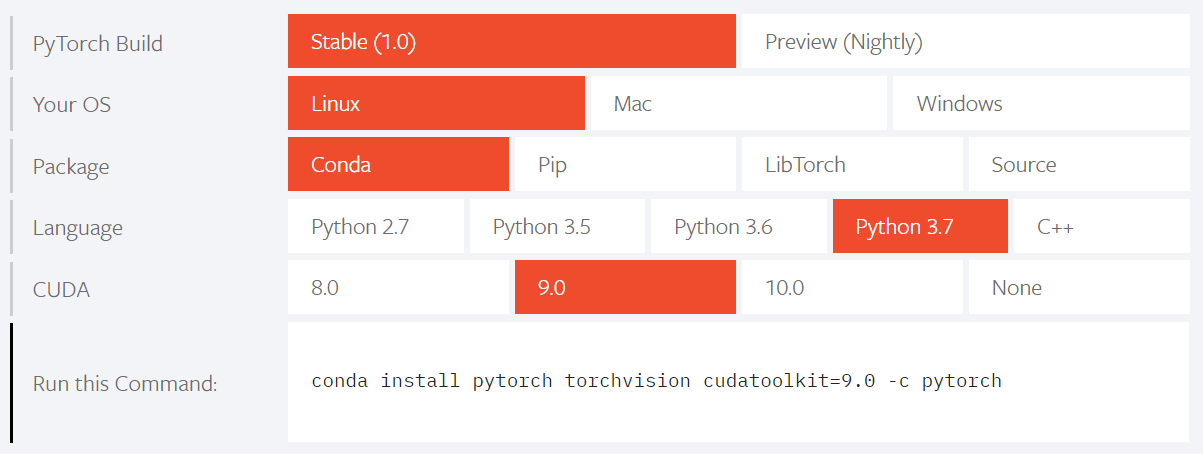1. Copy the given command at the end of the table and run it in your Terminal:
`\$ conda install pytorch torchvision cudatoolkit=9.0 -c pytorch`
1. This will install PyTorch 1.0 and torchvision

In the next section, we will show you how to verify the installation.

# Verifying the installation

Let's make sure that the installation is correct by importing PyTorch into Python:

1. Launch Python from a Terminal:
`# launch python from a terminal (linux/macos) or anaconda prompt (windows)\$ python>>>`
1. Import torch and get its version:
`# import PyTorch>>> import torch# get PyTorch version>>> torch.__version__'1.0.1.post2'`
1. Import torchvision and get its version:
`# import torchvision>>> import torchvision# get torchvision version>>> torchvision.__version__'0.2.2'`
1. Check if CUDA is available:
`# checking if cuda is available>>> torch.cuda.is_available()`
1. Get the number of CUDA devices:
`# get number of cuda/gpu devices>>> torch.cuda.device_count()1`
1. Get the CUDA device id:
`# get cuda/gpu device id>>> torch.cuda.current_device()0`
1. Get the CUDA device name:
`# get cuda/gpu device name>>> torch.cuda.get_device_name(0)'GeForce GTX TITAN X'`

In the next section, we will install other packages.

# Installing other packages

The majority of packages are installed using Anaconda. However, we may have to manually install other packages as we continue in this book:

1.  In this book, we will use Jupyter Notebook to implement our code. Install Jupyter Notebook in the environment:
`# install Jupyter Notebook in the conda environment\$ conda install -c anaconda jupyter`
1. Install matplotlib to show images and plots:
`# install matplotlib\$ conda install -c conda-forge matplotlib`
1. Install pandas to work with DataFrames:
`# install pandas \$ conda install -c anaconda pandas`

In the next section, we will explain each step in detail.

# How it works...

We started with the installation of Anaconda by following the necessary steps. After installing Anaconda, we created a conda environment for PyTorch experiments. You can use any operating system such as Windows, macOS, or Linux; we recommended Linux for this book. Next, we installed PyTorch in the conda environment.

Next, we verified the installation of PyTorch. We launched Python from a Terminal or Anaconda Prompt. Then, we imported torch and torchvision and obtained the package versions. Next, we checked the availability and number of CUDA devices. Our system is equipped with one GPU. Also, we got the CUDA device id and name. Our GPU device is GeForce GTX TITAN X. The default device ID is zero.

Finally, we installed Jupyter Notebook, matplotliband pandas in the conda environment. We will be developing the scripts in this book in Jupyter Notebook. We will also be using matplotlib to plot graphs and show images, as well as pandas to work with DataFrames.

# Working with PyTorch tensors

PyTorch is built on tensors. A PyTorch tensor is an n-dimensional array, similar to NumPy arrays.

If you are familiar with NumPy, you will see a similarity in the syntax when working with tensors, as shown in the following table:

 NumPy Arrays PyTorch tensors Description numpy.ones(.) torch.ones(.) Create an array of ones numpy.zeros(.) torch.zeros(.) Create an array of zeros numpy.random.rand(.) torch.rand(.) Create a random array numpy.array(.) torch.tensor(.) Create an array from given values x.shape x.shape or x.size() Get an array shape

In this recipe, you will learn how to define and change tensors, convert tensors into arrays, and move them between computing devices.

# How to do it...

For the following code examples, you can either launch Python or the Jupyter Notebook app from a Terminal.

# Defining the tensor data type

The default tensor data type is torch.float32. This is the most used data type for tensor operations. Let's take a look:

1. Define a tensor with a default data type:
`x = torch.ones(2, 2)print(x)print(x.dtype)tensor([[1., 1.], [1., 1.]])torch.float32`
1. Specify the data type when defining a tensor:
`# define a tensor with specific data typex = torch.ones(2, 2, dtype=torch.int8)print(x)print(x.dtype)tensor([[1, 1], [1, 1]], dtype=torch.int8)torch.int8`

In the next section, we will show you how to change the tensor's type.

# Changing the tensor's data type

We can change a tensor's data type using the .type method:

1. Define a tensor with the torch.uint8 type:
`x=torch.ones(1,dtype=torch.uint8)print(x.dtype)torch.uint8`

1. Change the tensor data type:
`x=x.type(torch.float)print(x.dtype)torch.float32`

In the next section, we will show you how to convert tensors into NumPy arrays.

# Converting tensors into NumPy arrays

We can easily convert PyTorch tensors into NumPy arrays. Let's take a look:

1. Define a tensor:
`x=torch.rand(2,2)print(x)print(x.dtype)tensor([[0.8074, 0.5728],        [0.2549, 0.2832]])torch.float32`
1. Convert the tensor into a NumPy array:
`y=x.numpy()print(y)print(y.dtype)[[0.80745    0.5727562 ] [0.25486636 0.28319395]] dtype('float32')`

In the next section, we will show you how to convert NumPy arrays into tensors.

# Converting NumPy arrays into tensors

We can also convert NumPy arrays into PyTorch tensors:

1. Define a NumPy array:
`import numpy as npx=np.zeros((2,2),dtype=np.float32)print(x)print(x.dtype)[[0. 0.] [0. 0.]]float32`
1. Convert the NumPy array into a PyTorch tensor:
`y=torch.from_numpy(x)print(y)print(y.dtype)tensor([[0., 0.],        [0., 0.]])torch.float32`

In the next section, we will show you how to move tensors between devices.

# Moving tensors between devices

By default, PyTorch tensors are stored on the CPU. PyTorch tensors can be utilized on a GPU to speed up computing. This is the main advantage of tensors compared to NumPy arrays. To get this advantage, we need to move the tensors to the CUDA device. We can move tensors onto any device using the .to method:

1. Define a tensor on CPU:
`x=torch.tensor([1.5, 2])print(x)print(x.device)x=torch.tensor([1.5, 2.])cpu`
1. Define a CUDA device:
`# define a cuda/gpu deviceif torch.cuda.is_available():    device = torch.device("cuda:0")`
1. Move the tensor onto the CUDA device:
`x = x.to(device) print(x)print(x.device)tensor([1.5, 2.], device='cuda:0')cuda:0`
1. Similarly, we can move tensors to CPU:
`# define a cpu devicedevice = torch.device("cpu")x = x.to(device) print(x)print(x.device)tensor([1.5, 2.])cpu`
1. We can also directly create a tensor on any device:
`# define a tensor on devicedevice = torch.device("cuda:0")x = torch.ones(2,2, device=device) print(x)tensor([[1., 1.],        [1., 1.]], device='cuda:0'`

In the next section, we will explain each step in detail.

# How it works...

First, we defined a tensor, obtained the tensor type, and changed its type. Then, we converted PyTorch tensors into NumPy arrays and vice versa. We also moved tensors between the CPU and CUDA devices. Next, we showed you how to change a tensor data type using the .type method. Then, we showed how to convert PyTorch tensors into NumPy arrays using the .numpy method.

After that, we showed you how to convert a NumPy array into a PyTorch tensor using the .from_numpy(x) method. Then, we showed you how to move tensors from a CPU device to a GPU device and vice versa, using the .to method. As you have seen, if you do not specify the device, the tensor will be hosted on the CPU device.

In most cases, it's assumed that we receive data in three groups: training, validation, and test. We use the training dataset to train the model. The validation dataset is used to track the model's performance during training. We use the test dataset for the final evaluation of the model. The target values of the test dataset are usually hidden from us. We need at least one training dataset and one validation dataset to be able to develop and train a model. Sometimes, we receive only one dataset. In such cases, we can split the dataset into two or three groups, as shown in the following diagram: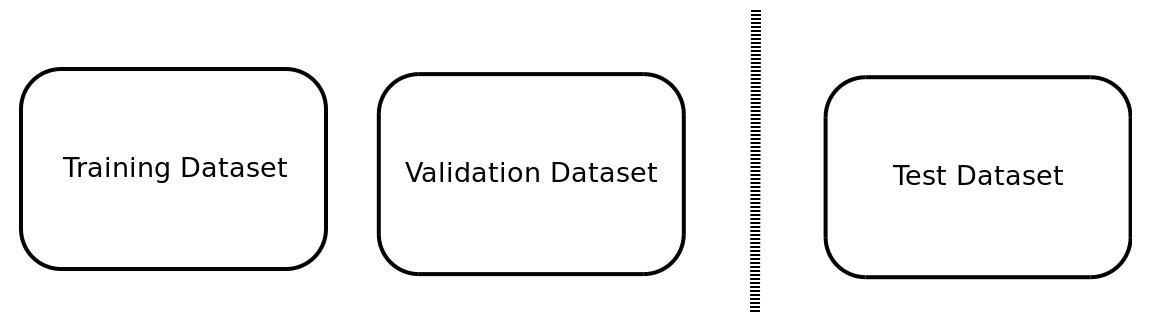Each dataset is comprised of inputs and targets. It is common to represent the inputs with x or X and the targets with y or Y. We add the suffixes train, valand test to distinguish each dataset.

In this recipe, we will learn about PyTorch data tools. We can use these tools to load and process data.

# How to do it...

In the following sections, you will learn how to use PyTorch packages to work with datasets.

The PyTorch torchvision package provides multiple popular datasets.

Let's load the MNIST dataset from torchvision:

1. First, we will load the MNIST training dataset:
```from torchvision import datasets

# path to store data and/or load from
path2data="./data"

1. Then, we will extract the input data and target labels:
```# extract data and targets
x_train, y_train=train_data.data,train_data.targets
print(x_train.shape)
print(y_train.shape)torch.Size([60000, 28, 28])torch.Size()```
1. Next, we will load the MNIST test dataset:
```# loading validation data
1. Then, we will extract the input data and target labels:
```# extract data and targets
x_val,y_val=val_data.data, val_data.targets
print(x_val.shape)
print(y_val.shape)torch.Size([10000, 28, 28])torch.Size()```

1. After that, we will add a new dimension to the tensors:
```# add a dimension to tensor to become B*C*H*W
if len(x_train.shape)==3:
x_train=x_train.unsqueeze(1)
print(x_train.shape)

if len(x_val.shape)==3:
x_val=x_val.unsqueeze(1)print(x_val.shape)torch.Size([60000, 1, 28, 28])torch.Size([10000, 1, 28, 28])```

Now, let's display a few sample images.

1. Next, we will import the required packages:
```from torchvision import utils
import matplotlib.pyplot as plt
import numpy as np
%matplotlib inline```
1. Then, we will define a helper function to display tensors as images:
```def show(img):
# convert tensor to numpy array
npimg = img.numpy()

# Convert to H*W*C shape
npimg_tr=np.transpose(npimg, (1,2,0))

plt.imshow(npimg_tr,interpolation='nearest')```
1. Next, we will create a grid of images and display them:
```# make a grid of 40 images, 8 images per row
print(x_grid.shape)

# call helper function
show(x_grid)```

The results are shown in the following image: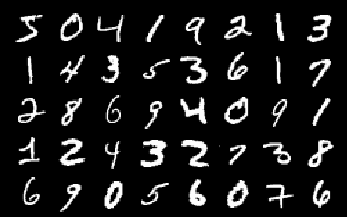In the next section, we will show you how to use data transformations together with a dataset.

# Data transformation

Image transformation (also called augmentation) is an effective technique that's used to improve a model's performance. The torchvision package provides common image transformations through the transform class. Let's take a look:

1. Let's define a transform class in order to apply some image transformations on the MNIST dataset:
`from torchvision import transforms# loading MNIST training datasettrain_data=datasets.MNIST(path2data, train=True, download=True)# define transformationsdata_transform = transforms.Compose([        transforms.RandomHorizontalFlip(p=1),        transforms.RandomVerticalFlip(p=1),        transforms.ToTensor(),    ])`
1. Let's apply the transformations on an image from the MNIST dataset:
`# get a sample image from training datasetimg = train_data# transform sample imageimg_tr=data_transform(img)# convert tensor to numpy arrayimg_tr_np=img_tr.numpy()# show original and transformed imagesplt.subplot(1,2,1)plt.imshow(img,cmap="gray")plt.title("original")plt.subplot(1,2,2)plt.imshow(img_tr_np,cmap="gray");plt.title("transformed")`

The results are shown in the following image: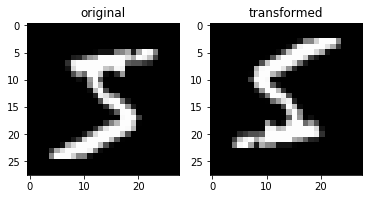1. We can also pass the transformer function to the dataset class:
`# define transformationsdata_transform = transforms.Compose([        transforms.RandomHorizontalFlip(1),        transforms.RandomVerticalFlip(1),        transforms.ToTensor(),    ])# Loading MNIST training data with on-the-fly transformationstrain_data=datasets.MNIST(path2data, train=True, download=True, transform=data_transform )`

In the next section, we will show you how to create a dataset from tensors.

# Wrapping tensors into a dataset

If your data is available in tensors, you can wrap them as a PyTorch dataset using the TensorDataset class. This will make it easier to iterate over data during training. Let's get started:

1. Let's create a PyTorch dataset by wrapping x_train and y_train :
```from torch.utils.data import TensorDataset

# wrap tensors into a dataset
train_ds = TensorDataset(x_train, y_train)
val_ds = TensorDataset(x_val, y_val)

for x,y in train_ds:
print(x.shape,y.item())
breaktorch.Size([1, 28, 28]) 5```

In the next section, we will show you how to define a data loader.

To easily iterate over the data during training, we can create a data loader using the DataLoader class, as follows:

1. Let's create two data loaders for the training and validation datasets:
```from torch.utils.data import DataLoader

# create a data loader from dataset

# iterate over batches
for xb,yb in train_dl:
print(xb.shape)
print(yb.shape)
break
torch.Size([8, 1, 28, 28])torch.Size()```

In the next section, we will explain each step in detail.

# How it works...

First, we imported the datasets package from torchvision. This package contains several famous datasets, including MNIST. Then, we downloaded the MNIST training dataset into a local folder. Once downloaded, you can set the download flag to False in future runs. Next, we extracted the input data and target labels into PyTorch tensors and printed their size. Here, the training dataset contains 60,000 inputs and targets. Then, we repeated the same step for the MNIST test dataset. To download the MNIST test dataset, we set the train flag to FalseHere, the test dataset contains 10,000 inputs and targets.

Next, we added a new dimension to the input tensors since we want the tensor shape to be B*C*H*W, where B, C, H, and W are batch size, channels, height, and width, respectively. This is the common shape for the inputs tensors in PyTorch. Then, we defined a helper function to display sample images. We used utils from torchvision to create a grid of 40 images in five rows and eight columns.

In the Data transformation subsection, we introduced the torchvision.transforms package. This package provides multiple transformation functions. We composed the RandomHorizontalFlip and RandomVerticalFlip methods to augment the dataset and the ToTensor method to convert images into PyTorch tensors. The probability of horizontal and vertical flips was set to p=1 to enforce flipping in the next step. We employed the data transformer on a sample image. Check out the original and the transformed image. The transformed image has been flipped both vertically and horizontally.

Then, we passed the transformer function to the dataset class. This way, data transformation will happen on-the-fly. This is a useful technique for large datasets that cannot be loaded into memory all at once.

In the Wrapping tensors into a dataset subsection, we created a dataset from tensors. For example, we can create a PyTorch dataset by wrapping x_train and y_train. This technique will be useful for cases where the input and output data is available as tensors.

In the Creating data loaders subsection, we used the DataLoader class to define data loaders. This is a good technique to easily iterate over datasets during training or evaluation. When creating a data loader, we need to specify the batch size. We created two data loaders from train_ds and val_ds. Then, we extracted a mini-batch from train_dl. Check out the shape of the mini-batch.

# Building models

A model is a collection of connected layers that process the inputs to generate the outputs. You can use the nn package to define models. The nn package is a collection of modules that provide common deep learning layers. A module or layer of nn receives input tensors, computes output tensors, and holds the weights, if any. There are two methods we can use to define models in PyTorch: nn.Sequential and nn.Module.

# How to do it...

We will define a linear layer, a two-layer network, and a multilayer convolutional network.

# Defining a linear layer

Let's create a linear layer and print out its output size:

`from torch import nn# input tensor dimension 64*1000input_tensor = torch.randn(64, 1000) # linear layer with 1000 inputs and 100 outputslinear_layer = nn.Linear(1000, 100) # output of the linear layeroutput = linear_layer(input_tensor) print(output.size())`

The following code will print out its output size:

`torch.Size([64, 100])`

In the next section, we will show you how to define a model using the nn.Sequential package.

# Defining models using nn.Sequential

We can use the nn.Sequential package to create a deep learning model by passing layers in order. Consider the two-layer neural network depicted in the following image: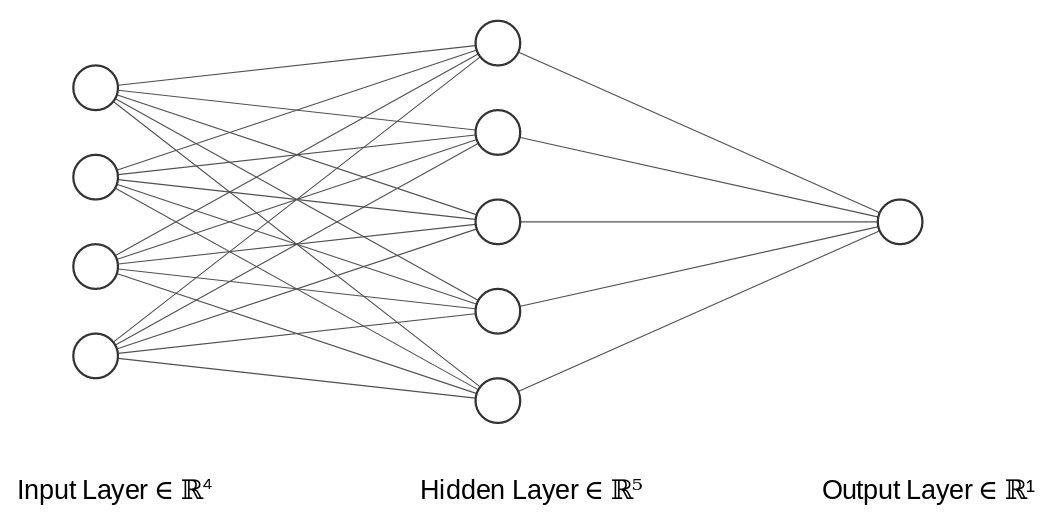As we can see, the network has four nodes as input, five nodes in the hidden layer, and one node as the output. Next, we will show you how to implement the network:

1. Let's implement and print the model using nn.Sequential :
`from torch import nn# define a two-layer modelmodel = nn.Sequential(    nn.Linear(4, 5),    nn.ReLU(),     nn.Linear(5, 1),)print(model)`

The output of the preceding code is as follows:

`Sequential(  (0): Linear(in_features=4, out_features=5, bias=True)  (1): ReLU()  (2): Linear(in_features=5, out_features=1, bias=True))`

In the next section, we will introduce another way of defining a model.

# Defining models using nn.Module

Another way of defining models in PyTorch is by subclassing the nn.Module class. In this method, we specify the layers in the __init__ method of the class. Then, in the forward method, we apply the layers to inputs. This method provides better flexibility for building customized models.

Consider a multilayer model, as shown in the following image: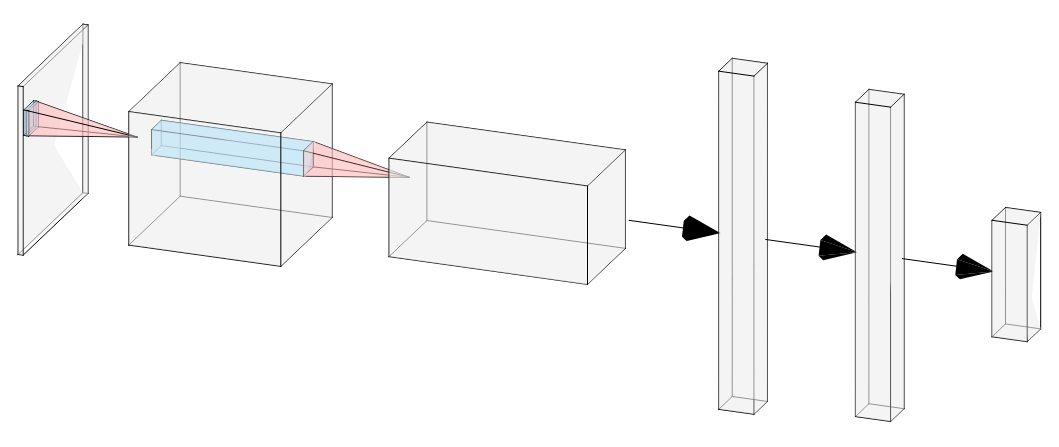As seen in the preceding image, the model has two convolutional layers and two fully connected layers. Next, we will show you how to implement the model.

Let's implement the multilayer model using nn.Module:

1. First, we will implement the bulk of the class:
`import torch.nn.functional as Fclass Net(nn.Module):    def __init__(self):        super(Net, self).__init__()    def forward(self, x):        pass`
1. Then, we will define the __init__ function:
`def __init__(self):    super(Net, self).__init__()    self.conv1 = nn.Conv2d(1, 20, 5, 1)    self.conv2 = nn.Conv2d(20, 50, 5, 1)    self.fc1 = nn.Linear(4*4*50, 500)    self.fc2 = nn.Linear(500, 10)`

1. Next, we will define the forward function:
`def forward(self, x):    x = F.relu(self.conv1(x))    x = F.max_pool2d(x, 2, 2)    x = F.relu(self.conv2(x))    x = F.max_pool2d(x, 2, 2)    x = x.view(-1, 4*4*50)    x = F.relu(self.fc1(x))    x = self.fc2(x)    return F.log_softmax(x, dim=1)`
1. Then, we will override both class functions, __init__ and forward:
`Net.__init__ = __init__Net.forward = forward`
1. Next, we will create an object of the Net class and print the model:
`model = Net()    print(model)`

The output of the preceding code is as follows:

`Net(  (conv1): Conv2d(1, 20, kernel_size=(5, 5), stride=(1, 1))  (conv2): Conv2d(20, 50, kernel_size=(5, 5), stride=(1, 1))  (fc1): Linear(in_features=800, out_features=500, bias=True)  (fc2): Linear(in_features=500, out_features=10, bias=True))`

In the next section, we will show you how to move the model to a CUDA device.

# Moving the model to a CUDA device

A model is a collection of parameters. By default, the model will be hosted on the CPU:

1. Let's get the model's device:
`print(next(model.parameters()).device)`

The preceding snippet will print the following output:

`cpu`
1. Then, we will move the model to the CUDA device:
`device = torch.device("cuda:0")model.to(device)print(next(model.parameters()).device)cuda:0`

In the next section, we will show you how to print the model summary.

# Printing the model summary

It is usually helpful to get a summary of the model to see the output shape and the number of parameters in each layer. Printing a model does not provide this kind of information. We can use the torchsummary package from the following GitHub repository for this purpose: https://github.com/sksq96/pytorch-summary. Let's get started:

1. Install the torchsummary package:
`pip install torchsummary`
1. Let's get the model summary using torchsummary:
`from torchsummary import summarysummary(model, input_size=(1, 28, 28))`

The preceding code will display the model summary when it's executed:

` ----------------------------------------------------------------         Layer (type)               Output Shape         Param # ================================================================             Conv2d-1           [-1, 20, 24, 24]             520             Conv2d-2             [-1, 50, 8, 8]          25,050             Linear-3                  [-1, 500]         400,500             Linear-4                   [-1, 10]           5,010 ================================================================ Total params: 431,080 Trainable params: 431,080 Non-trainable params: 0 ---------------------------------------------------------------- Input size (MB): 0.00 Forward/backward pass size (MB): 0.12 Params size (MB): 1.64 Estimated Total Size (MB): 1.76 ----------------------------------------------------------------`

In the next section, we will explain each step in detail.

# How it works...

First, we showed you how to create a linear layer using the nn package. The linear layer receives the input of the 64*1000 dimension, holds the weights of the 1000*100 dimension, and computes the output of the 64*100 dimension.

Next, we defined a two-layer neural network using nn.SequentialThere were four neurons in the input layer, five neurons in the hidden layer, and one neuron in the output layer. Using the print command, you can visualize the model's layers.

Next, we defined a multilayer model using nn.Module. The model has two Conv2d layers and two fully connected linear layers. For better code readability, we presented the Net class in a few snippets. First, we defined the bulk of the class. Then, we defined the __init__ function. As you saw, two Conv2d layers and two linear layers were defined in this function. Next, we defined the forward function. In this function, we defined the outline of the model and the way layers are connected to each other.

We used relu and max_pool2d from torch.nn.functional to define the activation function and pooling layers, respectively. Check out the way we used the .view method to flatten the extracted features from the Conv2d layers. The feature size was 4*4 and there were 50 channels in the self.conv2 layer. Due to this, the flatten size is 50*4*4. Also, check out the returned values from the forward function. As we saw, the log_softmax function was applied to the outputs. Next, we overrode the Net class functions. Finally, we created an object of the Net class and called it model. Then, we printed the model. Note that the print command does not show functional layers such as relu and max_pool2d

In the Moving the model to a CUDA device subsection, we verified that the model was hosted on the CPU device. Then, we moved the model to the CUDA device using the .to method. Here, we moved the first GPU or "cuda:0". If your system is equipped with multiple GPU devices, you can select a different number, for instance, "cuda:2".

Next, we installed the torchsummary package in the conda environment using the provided command.

If you do not want to install this package, the other option is to copy torchsummary.py into the folder of your code.

To get a model summary using torchsummary, we need to pass the input dimension to the summary function. For our MNIST example, we passed (1,28,28) as the input dimension and displayed the model summary. As seen, the output shape and the number of parameters of each layer, except functional layers, is shown in the summary.

Finally, we got the model summary using the torchsummary package.

# Defining the loss function and optimizer

The loss function computes the distance between the model outputs and targets. It is also called the objective function, cost function, or criterion. Depending on the problem, we will define the appropriate loss function. For instance, for classification problems, we usually define the cross-entropy loss.

We use the optimizer to update the model parameters (also called weights) during training. The optim package in PyTorch provides implementations of various optimization algorithms. These include stochastic gradient descent (SGD) and its variants, that is, Adam, RMSprop, and so on.

# How to do it...

In this section, we will look at defining the loss function and optimizer in PyTorch.

# Defining the loss function

We will define a loss function and test it on a mini-batch. Let's get started:

1. First, we will define the negative log-likelihood loss:
```from torch import nn
loss_func = nn.NLLLoss(reduction="sum")```
1. Let's test the loss function on a mini-batch:
```for xb, yb in train_dl:
# move batch to cuda device
xb=xb.type(torch.float).to(device)
yb=yb.to(device)
# get model output
out=model(xb)
# calculate loss value
loss = loss_func(out, yb)
print (loss.item())
break```

The preceding snippet will print the following output:

`69.37257385253906`
1. Let's compute the gradients with respect to the model parameters:
` # compute gradients loss.backward()`

In the next step, we will show you how to define an optimizer.

# Defining the optimizer

We will define the optimizer and present the steps backward. Let's get started:

1. Let's define the Adam optimizer:
`from torch import optimopt = optim.Adam(model.parameters(), lr=1e-4)`
1. Use the following code to update the model parameters:
` # update model parameters opt.step()`
1. Next, we set the gradients to zero:
` # set gradients to zero opt.zero_grad()`

In the next section, we will explain each step in detail.

# How it works...

First, we defined the loss function. We used the torch.nn package to define the negative log-likelihood loss. This loss is useful for training a classification problem with multiple classes. The input to this loss function should be log-probabilities. If you recall from the Building models section, we applied log_softmax at the output layer to get log-probabilities from the model. Next, we presented the forward path. We extracted a mini-batch, fed it to the model, and calculated the loss value. Next, we used the .backward method to compute the gradients of the loss with respect to the model parameters. This step will be used during the backpropagation algorithm.

Next, we define the Adam optimizer. The inputs to the optimizer are the model parameters and the learning rate. Then, we presented the .step() model to automatically update the model parameters. Don't forget to set the gradients to zero before computing the gradients of the next batch.

# Training and evaluation

Once all the ingredients are ready, we can start training the model. In this recipe, you will learn how to properly train and evaluate a deep learning model.

# How to do it...

We will develop helper functions for batch and epoch processing and training the model. Let's get started:

1. Let's develop a helper function to compute the loss value per mini-batch:
```def loss_batch(loss_func, xb, yb,yb_h, opt=None):
# obtain loss
loss = loss_func(yb_h, yb)

# obtain performance metric
metric_b = metrics_batch(yb,yb_h)

if opt is not None:
loss.backward()
opt.step()

return loss.item(), metric_b```
1. Next, we will define a helper function to compute the accuracy per mini-batch:
```def metrics_batch(target, output):
# obtain output class
pred = output.argmax(dim=1, keepdim=True)

# compare output class with target class
corrects=pred.eq(target.view_as(pred)).sum().item()
return corrects```
1. Next, we will define a helper function to compute the loss and metric values for a dataset:
```def loss_epoch(model,loss_func,dataset_dl,opt=None):
loss=0.0
metric=0.0
len_data=len(dataset_dl.dataset)
for xb, yb in dataset_dl:
xb=xb.type(torch.float).to(device)
yb=yb.to(device)

# obtain model output
yb_h=model(xb)

loss_b,metric_b=loss_batch(loss_func, xb, yb,yb_h, opt)
loss+=loss_b
if metric_b is not None:
metric+=metric_b
loss/=len_data
metric/=len_data
return loss, metric```
1. Finally, we will define the train_val function:
```def train_val(epochs, model, loss_func, opt, train_dl, val_dl):
for epoch in range(epochs):
model.train()
train_loss, train_metric=loss_epoch(model,loss_func,train_dl,opt)

model.eval()
val_loss, val_metric=loss_epoch(model,loss_func,val_dl)

accuracy=100*val_metric

print("epoch: %d, train loss: %.6f, val loss: %.6f, accuracy: %.2f" %(epoch, train_loss,val_loss,accuracy))```
1. Let's train the model for a few epochs:
```# call train_val function
num_epochs=5
train_val(num_epochs, model, loss_func, opt, train_dl, val_dl)```

Training will start and you should see its progress, as shown in the following code:

```epoch: 0, train loss: 0.294502, val loss: 0.093089, accuracy: 96.94
epoch: 1, train loss: 0.080617, val loss: 0.061121, accuracy: 98.06
epoch: 2, train loss: 0.050562, val loss: 0.049555, accuracy: 98.49
epoch: 3, train loss: 0.035071, val loss: 0.049693, accuracy: 98.45
epoch: 4, train loss: 0.025703, val loss: 0.050179, accuracy: 98.49```

In the next section, we will show you how to store and load a model.

Once training is complete, we'll want to store the trained parameters in a file for deployment and future use. There are two ways of doing so.

Let's look at the first method:

1. First, we will store the model parameters or state_dict in a file:
` # define path2weights path2weights="./models/weights.pt"  # store state_dict to file torch.save(model.state_dict(), path2weights)`
1. To load the model parameters from the file, we will define an object of the Net class:
`# define model: weights are randomly initiated_model = Net()`
1. Then, we will load state_dict from the file:
`weights=torch.load(path2weights)`
1. Next, we will set state_dict to the model:
`_model.load_state_dict(weights)`

Now, let's look at the second method:

1. First, we will store the model in a file:
` # define a path2model path2model="./models/model.pt"  # store model and weights into a file torch.save(model,path2model)`
1. To load the model parameters from the file, we will define an object of the Net class:
`# define model: weights are randomly initiated_model = Net()`
1. Then, we will load the model from the local file:
`_model=torch.load(path2model)`

In the next section, we will show you how to deploy the model.

# Deploying the model

To deploy a model, we need to load the model using the methods described in the previous section. Once the model has been loaded into memory, we can pass new data to the model. Let's get started:

1. To deploy the model on a sample image from the validation dataset, we will get a sample tensor:
```n=100
x= x_val[n]
y=y_val[n]
print(x.shape)
plt.imshow(x.numpy(),cmap="gray")torch.Size([1, 28, 28])```

The sample image is shown in the following screenshot: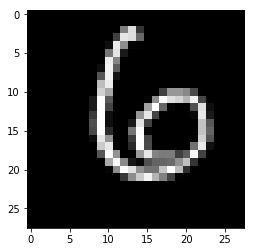1. Then, we will preprocess the tensor:
```# we use unsqueeze to expand dimensions to 1*C*H*W
x= x.unsqueeze(0)

# convert to torch.float32
x=x.type(torch.float)

# move to cuda device
x=x.to(device)```
1. Next, we will get the model prediction:
```# get model output
output=_model(x)

# get predicted class
pred = output.argmax(dim=1, keepdim=True)
print (pred.item(),y.item())```

The model prediction and the ground truth label are printed out after you execute the preceding code:

`6 6`

In the next section, we will explain each step in detail.

# How it works...

First, we developed a helper function to compute the loss and metric value per mini-batch. The opt argument of the function refers to the optimizer. If given, the gradients are computed and the model parameters are updated per mini-batch.

Next, we developed a helper function to compute a performance metric. The performance metric can be defined depending on the task. Here, we chose the accuracy metric for our classification task. We used output.argmax to get the predicted class with the highest probability.

Next, we defined a helper function to compute the loss and metric values for an entire dataset. We used the data loader object to get mini-batches, feed them to the model, and compute the loss and metrics per mini-batch. We used two running variables to add loss and metric values.

Next, we defined a helper function to train the model for multiple epochs. In each epoch, we also evaluated the model's performance using the validation dataset. Note that we set the model in training and evaluation modes using model.train() and model.eval(), respectively. Moreover, we used torch.no_grad() to stop autograd from calculating the gradients during evaluation.

Next, we explored two methods of storing the trained model. In the first method, we stored state_dict or model parameters only. Whenever we need the trained model for deployment, we have to create an object of the model, then load the parameters from the file, and then set the parameters to the model. This is the recommended method by PyTorch creators.

In the second method, we stored the model into a file. In other words, we stored both the model and state_dict into one file. Whenever we need the trained model for deployment, we need to create an object of the Net class. Then, we loaded the model from the file. So, there is no actual benefit of doing this compared to the previous method.

Next, we deployed the model on a sample image of the validation dataset. The sample image shape is C*H*W. Thus. we added a new dimension to become 1*C*H*WThen, we converted the tensor type into torch.float32 and moved it to a CUDA device.

Make sure that the model and data are hosted on the same device at deployment, otherwise, you will encounter an error.

# There's more...

Training deep learning models requires developing intuitions. We will introduce other techniques such as early stopping and learning rate schedules to avoid overfitting and improve performance in the next chapter.